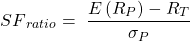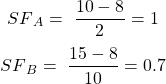101 Concepts for the Level I Exam

# Concept 10: Safety First RatioShortfall risk is the risk that portfolio’s return will fall below a specified minimum level of return over a given period of time.

Safety first ratio is used to measure shortfall risk. It is calculated as:A portfolio with higher safety first ratio is preferred over a portfolio with a lower safety first ratio.

An investor is considering two portfolios A and B. Portfolio A has an expected return of 10% and a standard deviation of 2%. Portfolio B has an expected return of 15% and a standard deviation of 10%. The minimum acceptable return for the investor is 8%. According to Roy’s safety first criteria, which portfolio should the investor select?

Solution:Since A has a higher safety first ratio, the investor should select portfolio A.

Roy’s safety first criteria states that an optimal portfolio minimizes the probability that the actual portfolio return will fall below the target return.